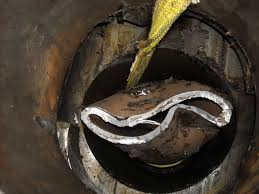# OIL & GAS CASING DESIGN - CASING COLLAPSE PRESSURE

## Casing Collapse Pressure CalculationsCasing collapse

### Introduction

The primary collapse loads are supplied by the column of fluid on the outside of the casing which act to collapse the pipe. These fluids are usually the mud and possibly the cement slurry in which the casing was set.

Since the column of mud increases with depth, collapse pressure is the highest at the bottom of the hole section and is zero at the surface.

The formula to calculate the hydrostatic pressure acting at a particular depth is:

Where
ρm is the density of the fluid in pcf.
h is depth in feet.

### Casing Collapse Pressure Calculations Design

The worst case design conditions are when the casing is void of fluid and the external force (collapse load) is the maximum mud weight when the casing was run.

In designing for collapse, the casing is assumed empty for surface casing, production casing and partially empty for intermediate casing.

Once the casing is cemented and the cement is set the cement acts to help increase the collapse resistance.

There are four formulas to calculate the collapse rating of casing (Pc) depending on the ratio of the pipe outer diameter to wall thickness.

#### Below we will have an example about how to run the casing collapse pressure calculations:

A string of 9-5/8” 53.5# L-80 casing is to be set in 75 pcf mud at a depth of 6000 ft.  Calculate the collapse rating for this casing, assume that the casing is empty. Then determine if the casing can safely be set to this depth in order to satisfy a safety factor for collapse of 1.125. Ym = 80,000 psi, ID = 8.535 in.

Solution:

Phyd = (75/144) x 6000 ft = 3,125 psi;

Since: De/t = 9.625 / 0.545 = 17.6605

We use Pc = Ym[A’/(D/t)-B’]-C

For N-80/L-80, A’ = 3.07; B’ = 0.0667; C = 1955

Pc = 80,000 [(3.07/17.6605) - 0.0667] - 1955 = 6616 psi                  SF = 6616 / 3125 = 2.12
hyd

Allowable Collapse Pressure = 6616 psi / 1.125 = 5880 psi.

Therefore from the previous casing collapse pressure calculations, the collapse load at 6000’ (3125 psi) is less than the allowable collapse (5880 psi), then it is safe to run.